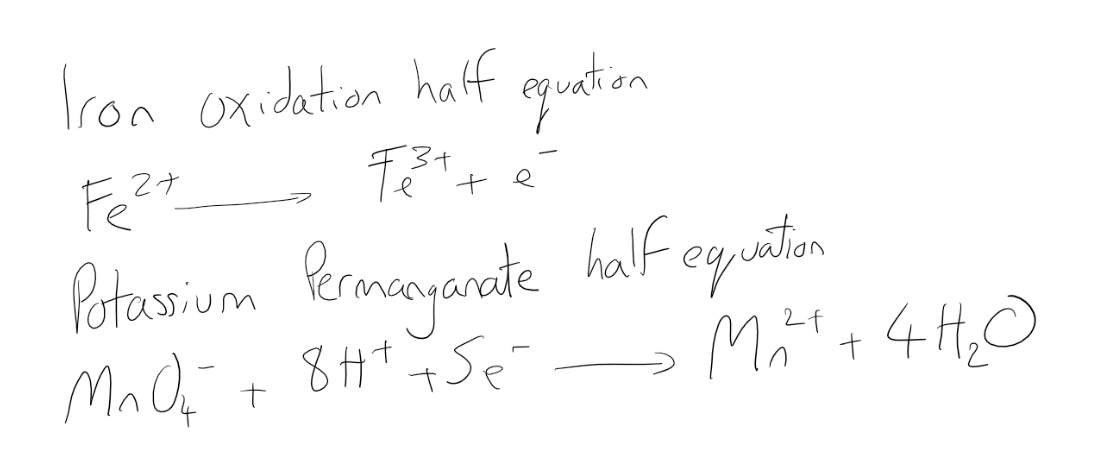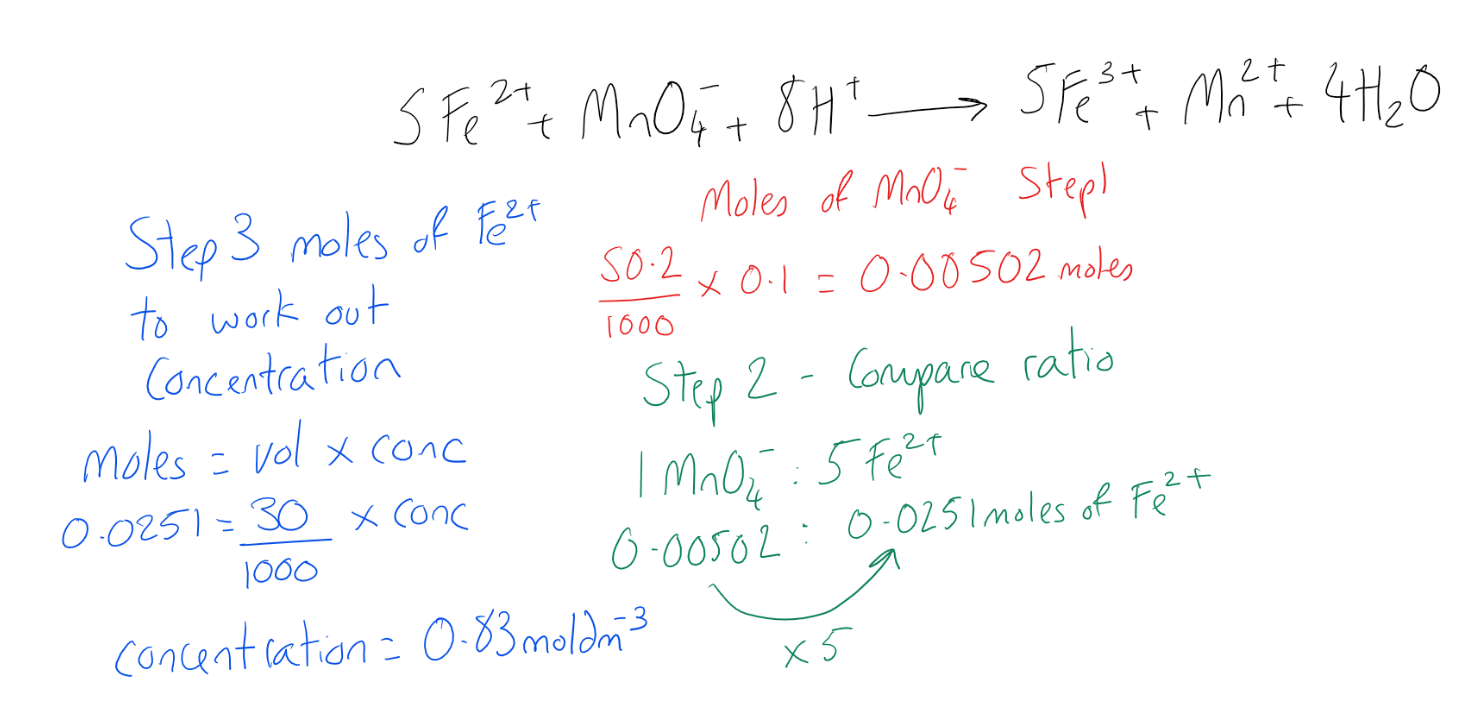Having trouble writing your personal statement?

MCAT Content / Titration / Redox Titrations

### Redox Titrations

Topic: Titration

Redox titrations are used to determine unknown amounts of a substance in a solution finding the equivalence point when the titrant and analyte have reacted stoichiometrically by transferring electrons.

As with acid-base titrations, a redox titration (also called an oxidation-reduction titration) can accurately determine the concentration of an unknown analyte by measuring it against a standardized titrant. Redox reactions are carried out in the same way as acid-base titrations using a burette and a known concentration of one reactant (titrant) and an unknown concentration of the other reactant (analyte). Sometimes an indicator is required, but many redox titrations have color changes that occur naturally due to the transfer of electrons.

The calculations carried out in a redox titration to determine the concentration of the analyte require a balanced equation developed from balancing half equations of the titrant and the analyte. In a redox titration, one reactant will oxidize itself producing electrons, thus acting as a reducing agent and the other reactant will accept these electrons, thus reducing itself acting as an oxidizing agent. The occurrence of both the process of oxidation and reduction results in a redox reaction.

For example in the titration of iron 2+ ions (Fe2+) with the oxidizing agent potassium permanganate (KMnO4) we can combine the half equations. Remember here that one of the equations has to show the gaining of electrons in reduction and one has to show the loss of electrons is oxidation. As the potassium permanganate is the oxidizing agent, we know it is reduced. This must mean the Fe2+ is oxidized and loses electrons.We can then balance this equation by ensuring the same number of electrons in each equation so when combined they cancel out on either side of the equation.Now we have a balanced equation. We can use this stoichiometric equation to calculate unknown concentrations and volumes from data collected in the titration. Expanding on this example, if we wanted to know the concentration of iron sulphate in a 30cm3 sample when it required 50.2cm3 of potassium permanganate with a concentration of 0.1mol dm-3 we can work this out using the equation moles = concentration (mol dm-3) x volume (dm3) compare the molar ratio of iron to potassium permanganate and solve for the unknown concentration of Fe2+ ions in solution.Key Points

• The titrant is the standardized solution; the analyte is the analyzed substance.

• Redox titration determines the concentration of an unknown solution (analyte) that contains an oxidizing or reducing agent.

• Not all titrations require an external indicator. Some titrants can serve as their own indicators, such as when potassium permanganate is titrated against a colorless analyte.

• Remember, an oxidizing agent oxidizes something else. Oxidation is loss of electrons (OIL RIG). Therefore, an oxidizing agent takes electrons from that other substance and must gain electrons.

Key Terms

Redox titration/oxidation-reduction titration: A type of titration based on a redox reaction between the analyte and titrant.

Titrant: The acid or base in the burette that has a known concentration

Analyte: The acid or base which has an unknown concentration

Burette: An accurate piece of equipment used for adding small volumes of a solution to another solution

Oxidation: Oxidation occurs when an atom, molecule, or ion loses one or more electrons in a chemical reaction. When oxidation occurs, the oxidation state of the chemical species increases.

Reduction: A chemical reaction that involves the gaining of electrons by one of the atoms involved in the reaction between two chemicals.

Reducing agent: A reactant that undergoes oxidation in order to produce electrons in a reaction

Oxidizing agent: A reactant that undergoes a reduction in a reaction in order to gain electrons

Billing Information